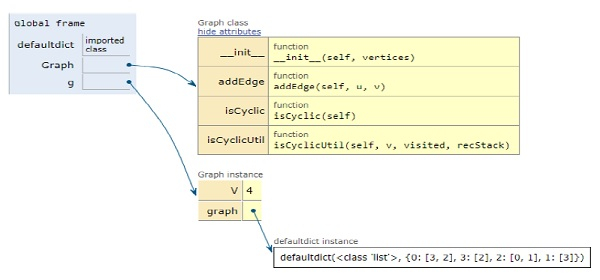# Python Program for Detect Cycle in a Directed Graph

Problem statement − We are given a directed graph, we need to check whether the graph contains a cycle or not. The output should be true if the given graph contains at least one cycle, otherwise false.

Now let’s observe the solution in the implementation below −

## Example

Live Demo

# collections module
from collections import defaultdict
# class for creation of graphs
class Graph():
# constructor
def __init__(self, vertices):
self.graph = defaultdict(list)
self.V = vertices
self.graph[u].append(v)
def isCyclicUtil(self, v, visited, recStack):
# Marking current node visited and addition to recursion stack
visited[v] = True
recStack[v] = True
# if any neighbour is visited and in recStack then graph is cyclic in nature
for neighbour in self.graph[v]:
if visited[neighbour] == False:
if self.isCyclicUtil(neighbour, visited, recStack) == True:
return True
elif recStack[neighbour] == True:
return True
# pop the node after the end of recursion
recStack[v] = False
return False
# Returns true if graph is cyclic
def isCyclic(self):
visited = [False] * self.V
recStack = [False] * self.V
for node in range(self.V):
if visited[node] == False:
if self.isCyclicUtil(node, visited, recStack) == True:
return True
return False
g = Graph(4)
if g.isCyclic() == 1:
print ("Graph is cyclic in nature")
else:
print ("Graph is non-cyclic in nature")

## Output

Graph is cyclic in natureAll the variables are declared in the local scope and their references are seen in the figure above.

## Conclusion

In this article, we have learned about how we can make a Python Program to Detect Cycle in a Directed Graph